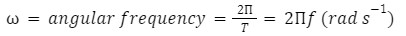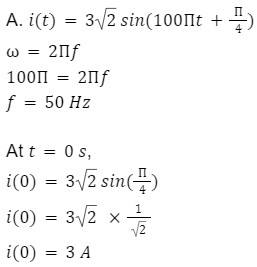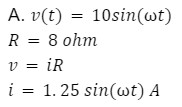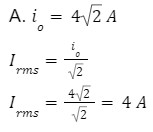• Call Now

1800-102-2727•

# Alternating current, AC Vs DC, Sinusoidal AC, practice problems, FAQs

Alternating current (AC) is less expensive and easy to generate than DC. Most of the household electrical appliances use AC such as fan, cooler, air conditioner, etc. On the other hand few electronic appliances use DC such as cellphones. In this article we will be discussing alternating current in detail. We will also discuss the differences between AC and DC.

## Story behind ACThe story behind AC is the Story that powered the world. In the late 19th century in New York, something incredible was happening at the Edison electric co. The famous Thomas Edison was trying to create an inexpensive and efficient light source, which could replace gas lanterns. After more than 100 failed attempts, the use of carbonized thread provided the breakthrough. He had invented a compact electric bulb which could glow for a sustained period of time. Edison was a proponent of DC which was the prevalent form of electric current at that time.

The DC or direct current is unidirectional in an electrical circuit i.e the current flows in a particular direction in the circuit. At the same time when Edison discovered the electric bulb, a young student by the name of Nicola Tesla came up with a different vision. He believed that AC is the more efficient form of current.

The AC current is the current which changes its direction periodically after a certain period of time. Although Edison had already invented the light bulb, it wasn’t enough for household usage. For every household to use the electric bulb, they required distribution of electricity at every household. Thus, Edison started working on establishing the world’s first dc power station named “Pearl street station”. The battle between AC vs DC has started. The pivotal battle of the current is won by Tesla’s AC.

## Principle of Generation of AC

A coil of conducting wire is rotated in a high magnetic field to generate alternating voltage. The magnetic flux linked with the coil changes with time and an alternating emf is thus induced. Instantaneous flux linked with coil is=(AB)n

=ABn cos(t+0)

Where, A=area of the coil (m2)

B=magnetic field (Tesla)

n=number of turnsf=frequency in Hertz

0=initial phase angle

With the change of time cos(t+0) changes consequently as EMF V is induced. According to Faraday’s lawHere, Vm=Voltage amplitude of sinusoidal voltage or peak value of AC voltage

Where, Vm=ABn

## Alternating Current

The currents whose direction changes periodically through a load are known as alternating currents and the voltage is known as alternating voltage. Most of the electrical devices require AC voltage because electrical energy in AC form can be easily transmitted over long distances without much loss. AC voltages can be easily converted to other voltages by step up or step down transformers.

In case of periodic current or voltage, they are said to be alternating if:

• Its amplitude is constant
• Alternative half cycle is positive and half negativeAs shown in the first diagram damped oscillations are not AC because their amplitude is not constant. Ihe second diagram amplitude is not oscillating between positive and negative cycles so it is also not AC.

If the currents or voltages vary periodically as “sin” or “cos” function of time, the current or voltage is said to be sinusoidal, as shown in the figure below.We use the term AC source for any device that supplied a sinusoidally varying voltage (potential difference) v or current i. The usual circuit diagram symbol for AC source is shown in figure below.The sinusoidal voltage can be described by following function such as,

v=V0cos(t) or v=V0sin(t)

In the above expression, v is the instantaneous potential difference, Vo is the maximum potential difference which we call the voltage amplitude. is angular frequency (rad s-1) which is equal to =2f.Similarly, i=I0cos(t) or i=I0sin(t)

Where, i is instantaneous current and Io is the maximum or current amplitude.

## AC vs DC

Let’s see the difference between AC and DC.

 Alternating Current (AC) Direct Current (DC) AC is safe to be transferred over a longer distance even between two cities and can maintain the electric power. DC cannot travel for a very long distance. It loses electric power. The rotating magnets cause the change in direction of electric flow. The steady magnetism makes DC flow in a single direction. Depending on the country, the frequency of AC varies. But, generally, the frequency is 50 Hz or 60 Hz. DC has no frequency or zero frequency. In AC the flow of current changes its direction forward and backward periodically. It flows in a single direction steadily. We can not store AC using batteries or cells We can store DC using batteries or cells

## Practice Problems

Q 1. An alternating current is given by the equation i(t)=32 sin(100t+4) A. Find the frequency of AC and value of current at t=0 s?Q 2. Find the current flowing through the resistor, R=8 ohm if applied AC voltage is v(t)=10sin(t) V?Q 3. Frequency of alternating current is 50 Hz. Then in how much time does it reverse its direction?

T=1f=150

Time to reverse the direction is half of the time period.

So it reverse its direction in 1100 seconds.

Q 4. Peak value of AC current is 42 A. Find the RMS value of current?## FAQs

Q 1. What are the benefits of using AC instead of DC?
AC is less expensive and easy to generate than DC. The distance covered by AC is more than that of the DC. The power loss during transmission in AC is less when compared to DC.

Q 2. How can we convert DC into AC?
An inverter converts the DC electricity from sources such as batteries or fuel cells to AC electricity. So we can use an inverter to convert DC into AC.

Q 3. What is the device used to convert AC into DC?
Rectifiers are used to convert alternating current into direct current.

Q 4. Why can AC not be stored?
It's crucial to realise that batteries don't store energy directly in them. Electrical energy is stored in the form of chemical energy. The positive connection of an AC source is linked to the battery's positive terminal, while the negative terminal is connected to the battery's negative terminal. The current begins to move. However, AC reverses polarity, and there is no true energy supply. Because the positive half cycle cancels out the negative half cycle, this is the case. If this procedure continues, the battery may be harmed. As a result, AC cannot be stored in batteries.Talk to our expert
Resend OTP Timer =
By submitting up, I agree to receive all the Whatsapp communication on my registered number and Aakash terms and conditions and privacy policy